# RD Sharma Solutions for Class 7 Maths Chapter 22 Data Handling - I (Collection and Organisation of Data)

The PDF of RD Sharma Solutions for Class 7 Maths Chapter 22 Data Handling – I (Collection and Organisation of Data) are downloaded from the given links. The solutions are prepared by BYJU’S experts in Maths. The RD Sharma Solutions for exercise wise problems are prepared after vast research is conducted on each topic. The step-wise solutions prepared by subject experts help students to solve problems with competence.

Chapter 22, Data handling – I (Collection and Organisation of Data) is based on observation and collection of data. RD Sharma Solutions for Class 7 includes answers to all questions present in this exercise. Let us have a look at some of the concepts that are being discussed in this Chapter.

• Primary data
• Secondary data
• Organisation of data
• Frequency distribution
• Construction of frequency distribution

## Download the PDF of RD Sharma Solutions For Class 7 Maths Chapter 22 Data Handling – I (Collection and Organisation of Data)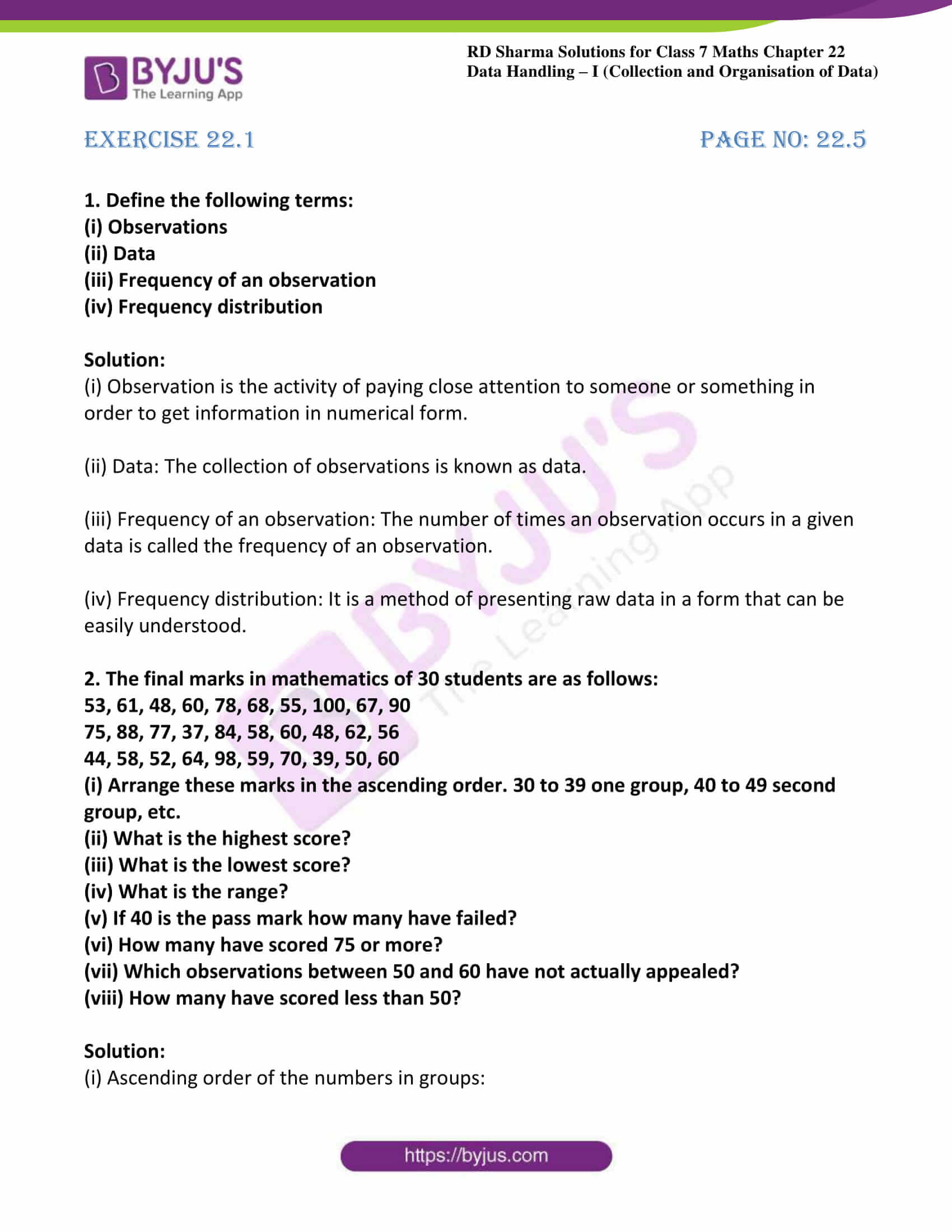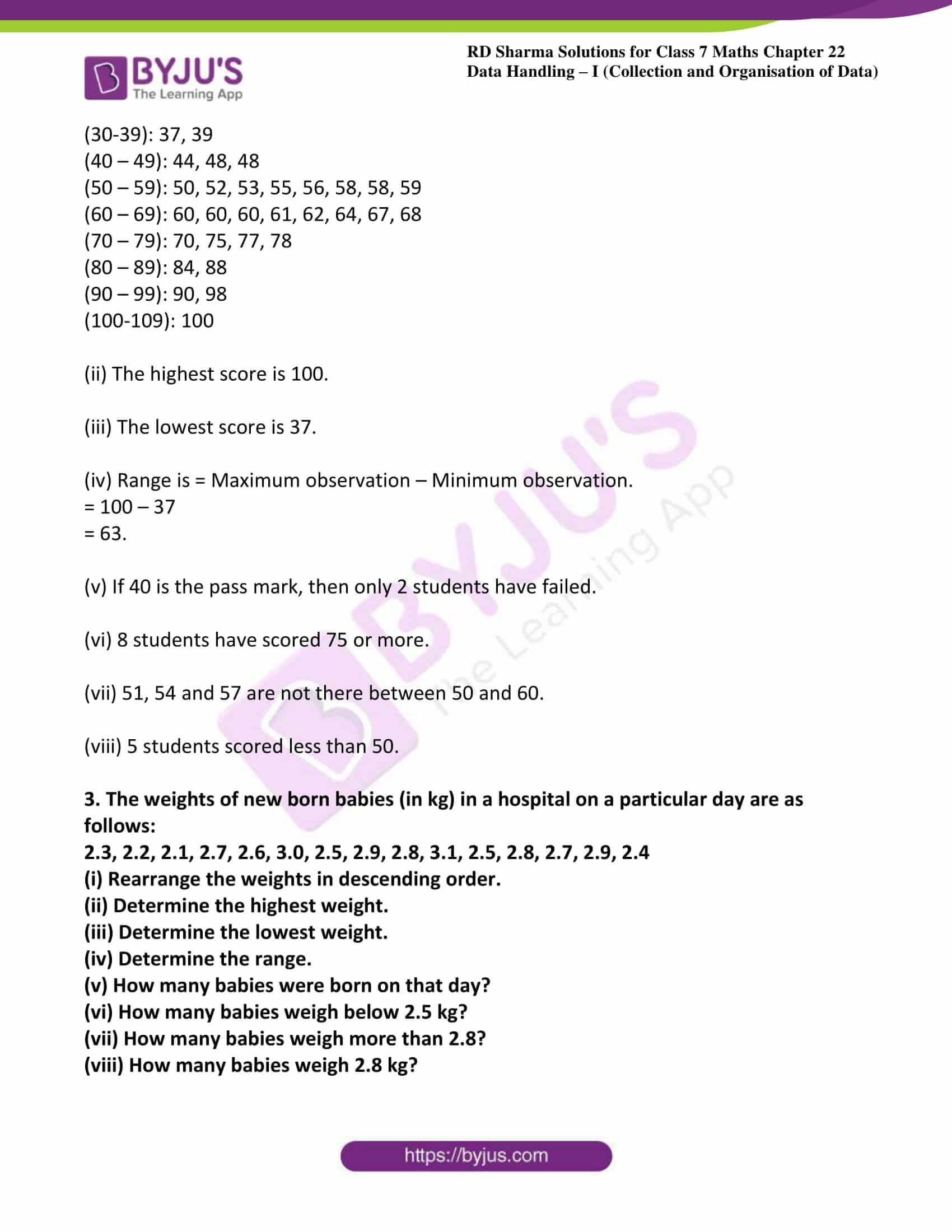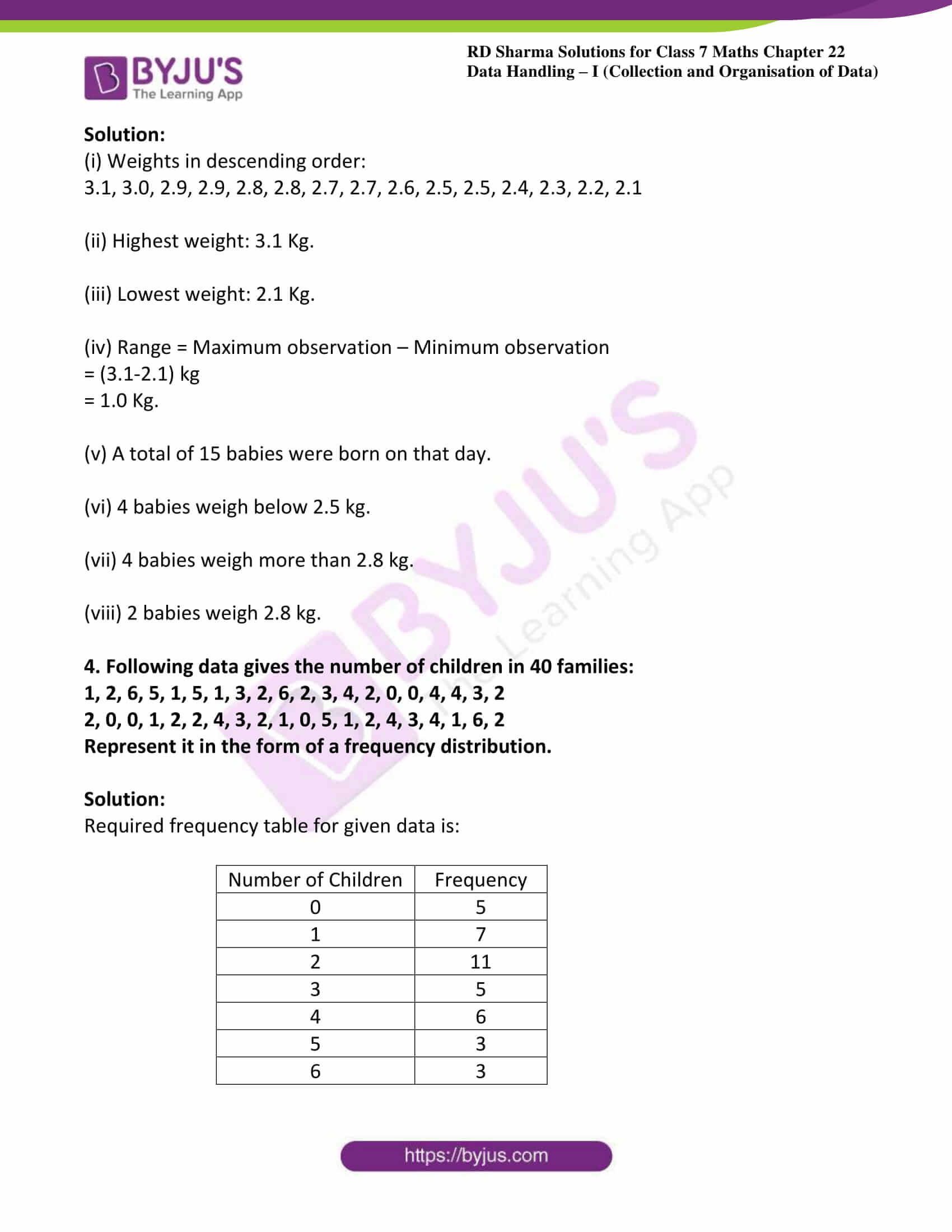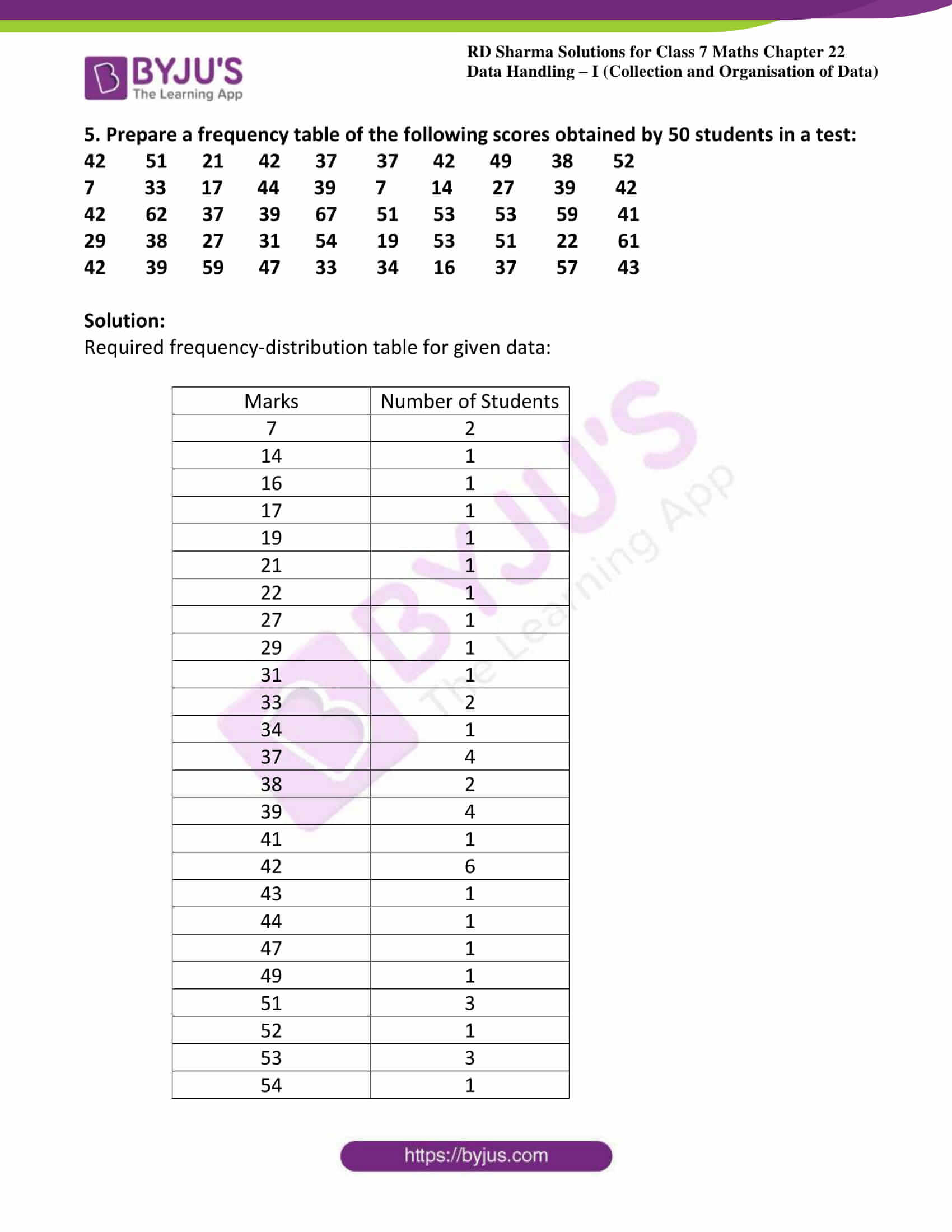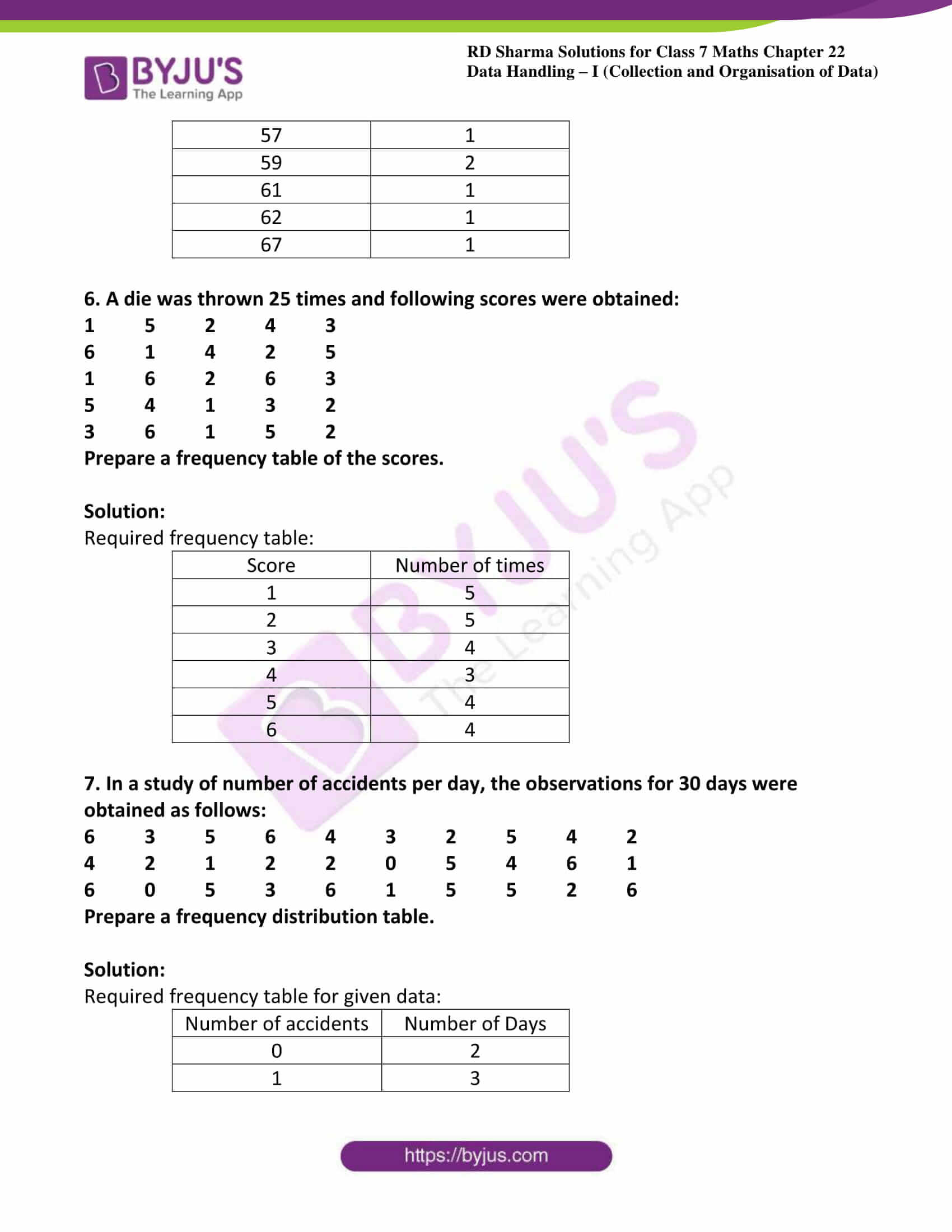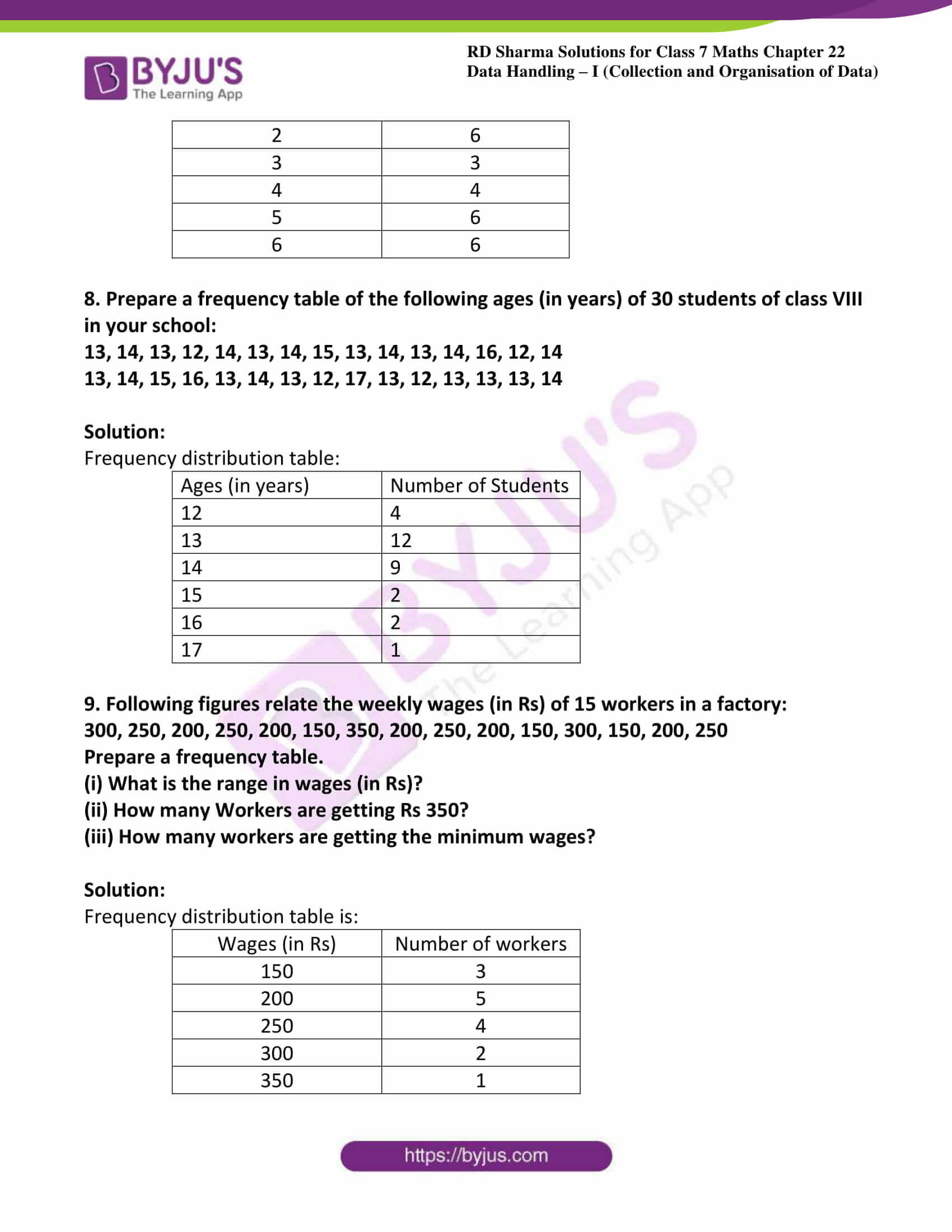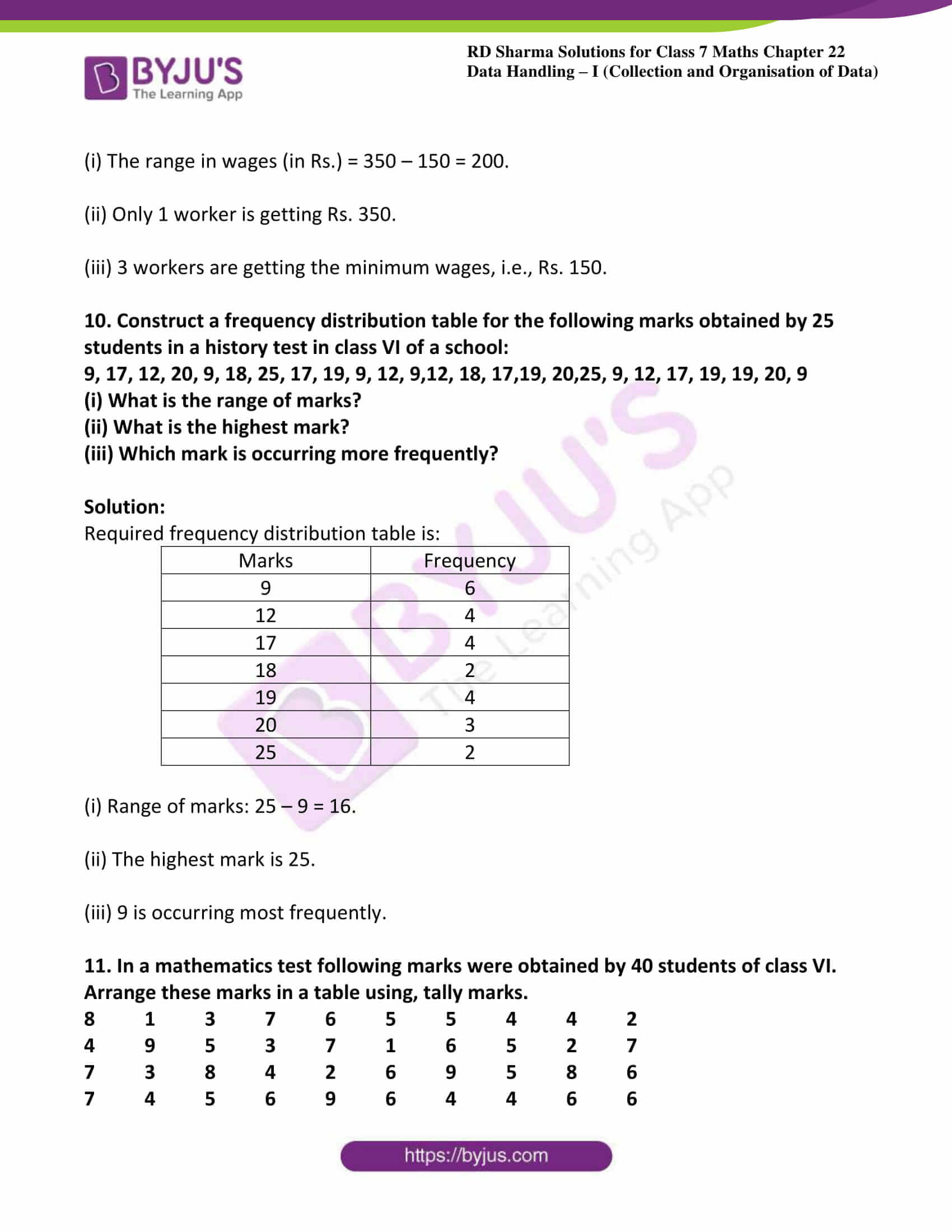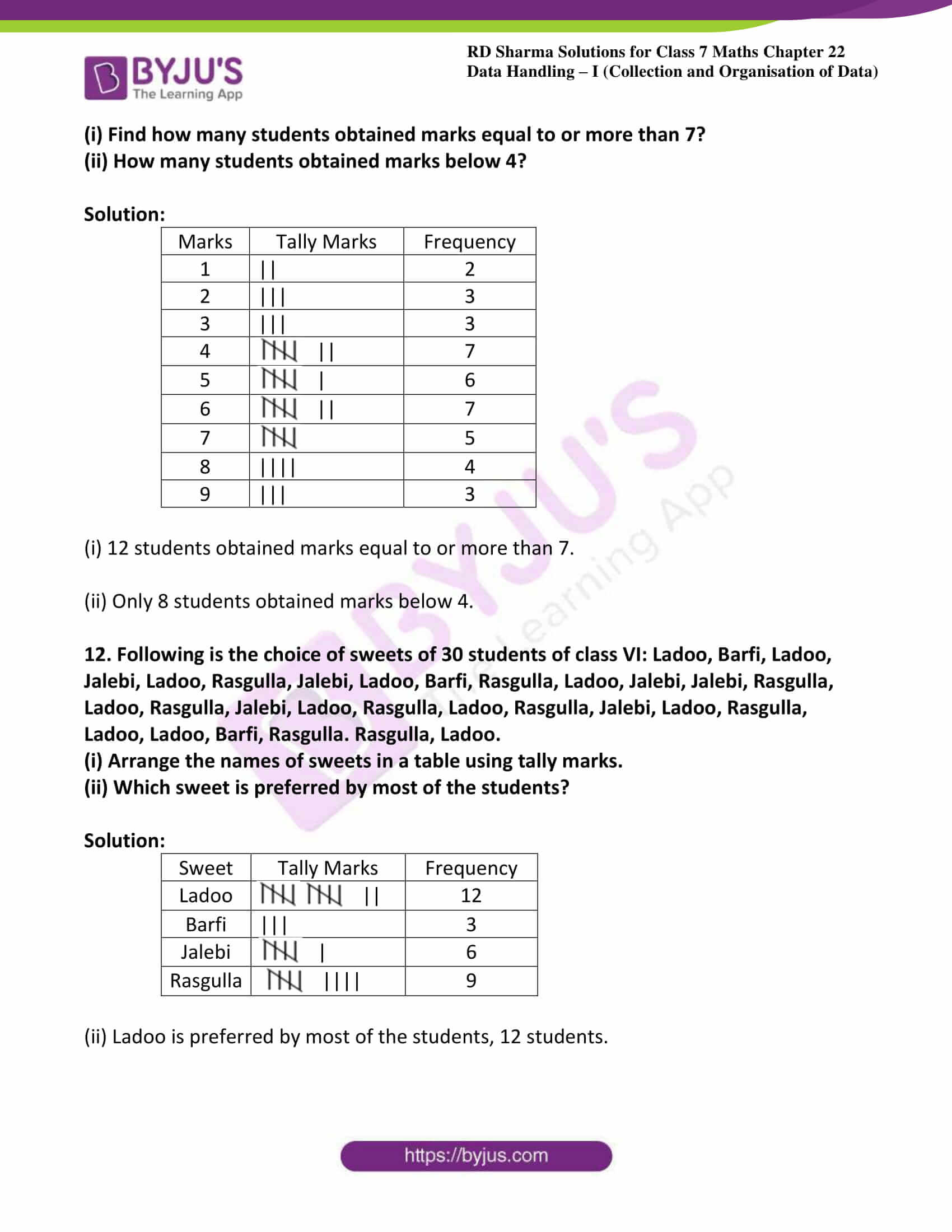### Access answers to Maths RD Sharma Solutions For Class 7 Chapter 22 – Data Handling – I (Collection and Organisation of Data)

1. Define the following terms:

(i) Observations

(ii) Data

(iii) Frequency of an observation

(iv) Frequency distribution

Solution:

(i) Observation is the activity of paying close attention to someone or something in order to get information in numerical form.

(ii) Data: The collection of observations is known as data.

(iii) Frequency of an observation: The number of times an observation occurs in a given data is called the frequency of an observation.

(iv) Frequency distribution: It is a method of presenting raw data in a form that can be easily understood.

2. The final marks in mathematics of 30 students are as follows:

53, 61, 48, 60, 78, 68, 55, 100, 67, 90

75, 88, 77, 37, 84, 58, 60, 48, 62, 56

44, 58, 52, 64, 98, 59, 70, 39, 50, 60

(i) Arrange these marks in the ascending order. 30 to 39 one group, 40 to 49 second group, etc.

(ii) What is the highest score?

(iii) What is the lowest score?

(iv) What is the range?

(v) If 40 is the pass mark how many have failed?

(vi) How many have scored 75 or more?

(vii) Which observations between 50 and 60 have not actually appealed?

(viii) How many have scored less than 50?

Solution:

(i) Ascending order of the numbers in groups:

(30-39): 37, 39

(40 – 49): 44, 48, 48

(50 – 59): 50, 52, 53, 55, 56, 58, 58, 59

(60 – 69): 60, 60, 60, 61, 62, 64, 67, 68

(70 – 79): 70, 75, 77, 78

(80 – 89): 84, 88

(90 – 99): 90, 98

(100-109): 100

(ii) The highest score is 100.

(iii) The lowest score is 37.

(iv) Range is = Maximum observation – Minimum observation.

= 100 – 37

= 63.

(v) If 40 is the pass mark, then only 2 students have failed.

(vi) 8 students have scored 75 or more.

(vii) 51, 54 and 57 are not there between 50 and 60.

(viii) 5 students scored less than 50.

3. The weights of new born babies (in kg) in a hospital on a particular day are as follows:

2.3, 2.2, 2.1, 2.7, 2.6, 3.0, 2.5, 2.9, 2.8, 3.1, 2.5, 2.8, 2.7, 2.9, 2.4

(i) Rearrange the weights in descending order.

(ii) Determine the highest weight.

(iii) Determine the lowest weight.

(iv) Determine the range.

(v) How many babies were born on that day?

(vi) How many babies weigh below 2.5 kg?

(vii) How many babies weigh more than 2.8?

(viii) How many babies weigh 2.8 kg?

Solution:

(i) Weights in descending order:

3.1, 3.0, 2.9, 2.9, 2.8, 2.8, 2.7, 2.7, 2.6, 2.5, 2.5, 2.4, 2.3, 2.2, 2.1

(ii) Highest weight: 3.1 Kg.

(iii) Lowest weight: 2.1 Kg.

(iv) Range = Maximum observation – Minimum observation

= (3.1-2.1) kg

= 1.0 Kg.

(v) A total of 15 babies were born on that day.

(vi) 4 babies weigh below 2.5 kg.

(vii) 4 babies weigh more than 2.8 kg.

(viii) 2 babies weigh 2.8 kg.

4. Following data gives the number of children in 40 families:

1, 2, 6, 5, 1, 5, 1, 3, 2, 6, 2, 3, 4, 2, 0, 0, 4, 4, 3, 2

2, 0, 0, 1, 2, 2, 4, 3, 2, 1, 0, 5, 1, 2, 4, 3, 4, 1, 6, 2

Represent it in the form of a frequency distribution.

Solution:

Required frequency table for given data is:

 Number of Children Frequency 0 5 1 7 2 11 3 5 4 6 5 3 6 3

5. Prepare a frequency table of the following scores obtained by 50 students in a test:

42        51       21       42       37       37       42       49       38       52

7          33       17       44       39      7         14        27        39        42

42        62       37       39       67       51       53        53        59        41

29        38       27       31       54        19       53        51        22        61

42        39       59       47       33        34       16        37        57        43

Solution:

Required frequency-distribution table for given data:

 Marks Number of Students 7 2 14 1 16 1 17 1 19 1 21 1 22 1 27 1 29 1 31 1 33 2 34 1 37 4 38 2 39 4 41 1 42 6 43 1 44 1 47 1 49 1 51 3 52 1 53 3 54 1 57 1 59 2 61 1 62 1 67 1

6. A die was thrown 25 times and following scores were obtained:

1          5          2          4          3

6          1          4          2          5

1          6          2          6          3

5          4          1          3          2

3          6          1          5          2

Prepare a frequency table of the scores.

Solution:

Required frequency table:

 Score Number of times 1 5 2 5 3 4 4 3 5 4 6 4

7. In a study of number of accidents per day, the observations for 30 days were obtained as follows:

6          3          5          6          4          3          2          5          4          2

4          2          1          2          2          0          5          4          6          1

6          0          5          3          6          1          5          5          2          6

Prepare a frequency distribution table.

Solution:

Required frequency table for given data:

 Number of accidents Number of Days 0 2 1 3 2 6 3 3 4 4 5 6 6 6

8. Prepare a frequency table of the following ages (in years) of 30 students of class VIII in your school:

13, 14, 13, 12, 14, 13, 14, 15, 13, 14, 13, 14, 16, 12, 14

13, 14, 15, 16, 13, 14, 13, 12, 17, 13, 12, 13, 13, 13, 14

Solution:

Frequency distribution table:

 Ages (in years) Number of Students 12 4 13 12 14 9 15 2 16 2 17 1

9. Following figures relate the weekly wages (in Rs) of 15 workers in a factory:

300, 250, 200, 250, 200, 150, 350, 200, 250, 200, 150, 300, 150, 200, 250

Prepare a frequency table.

(i) What is the range in wages (in Rs)?

(ii) How many Workers are getting Rs 350?

(iii) How many workers are getting the minimum wages?

Solution:

Frequency distribution table is:

 Wages (in Rs) Number of workers 150 3 200 5 250 4 300 2 350 1

(i) The range in wages (in Rs.) = 350 – 150 = 200.

(ii) Only 1 worker is getting Rs. 350.

(iii) 3 workers are getting the minimum wages, i.e., Rs. 150.

10. Construct a frequency distribution table for the following marks obtained by 25 students in a history test in class VI of a school:

9, 17, 12, 20, 9, 18, 25, 17, 19, 9, 12, 9,12, 18, 17,19, 20,25, 9, 12, 17, 19, 19, 20, 9

(i) What is the range of marks?

(ii) What is the highest mark?

(iii) Which mark is occurring more frequently?

Solution:

Required frequency distribution table is:

 Marks Frequency 9 6 12 4 17 4 18 2 19 4 20 3 25 2

(i) Range of marks: 25 – 9 = 16.

(ii) The highest mark is 25.

(iii) 9 is occurring most frequently.

11. In a mathematics test following marks were obtained by 40 students of class VI. Arrange these marks in a table using, tally marks.

8          1          3          7          6          5          5          4          4          2

4          9          5          3          7          1          6          5          2          7

7          3          8          4          2          6          9          5          8          6

7          4          5          6          9          6          4          4          6          6

(i) Find how many students obtained marks equal to or more than 7?

(ii) How many students obtained marks below 4?

Solution:

 Marks Tally Marks Frequency 1 || 2 2 ||| 3 3 ||| 3 4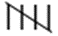|| 7 5| 6 6|| 7 75 8 |||| 4 9 ||| 3

(i) 12 students obtained marks equal to or more than 7.

(ii) Only 8 students obtained marks below 4.

 Sweet Tally Marks Frequency Ladoo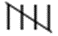|| 12 Barfi ||| 3 Jalebi| 6 Rasgulla|||| 9